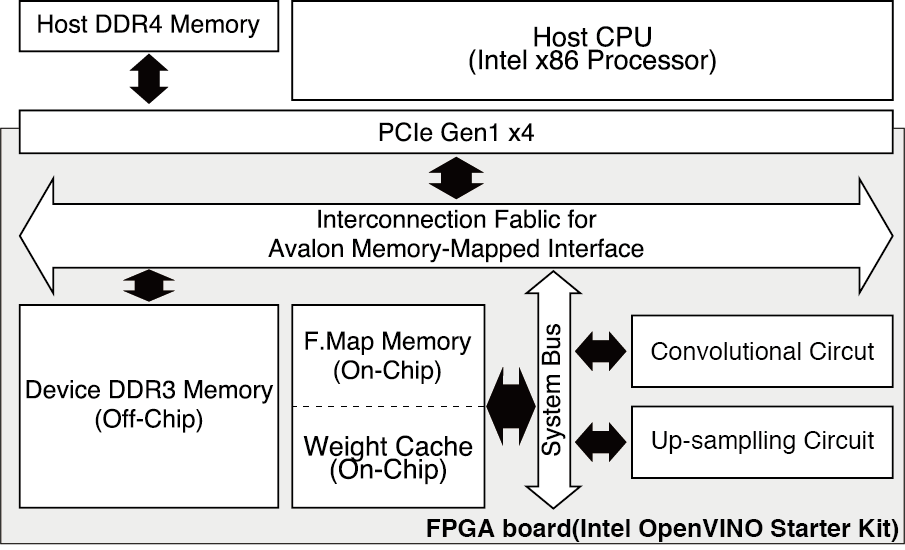﻿ InnovateFPGA | APJ | AP016 - Realtime Monocular Depth Estimator
Annual: 2019

📁Machine Learning
(Tokyo Institute of Technology)
📅Oct 15, 2019👀 3434   💬 3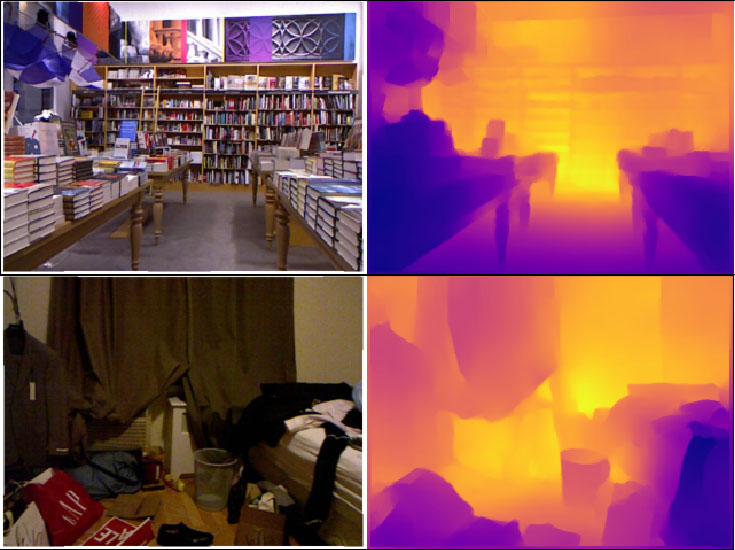AP016 » Realtime Monocular Depth Estimator

### Description

This project is an FPGA implementation of the accurate monocular depth estimator with realtime. The monocular depth estimation estimates the depth from single RGB images. Estimating depth is important to understand the scene and it improves the performance of 3D object detections and semantic segmentations. Also, there is many applications requiring depth estimation such as robotics, 3D modeling and driving automation systems. The monocular depth estimation is extremely effective in these applications where the stereo images, optical flow, or point clouds cannot be used. Moreover, there is the possibility to replace an expensive radar sensor into the general RGB camera.

We choose the CNN (Convolutional Neural Network)-based monocular depth estimation since the stereo monocular estimation requires larger resource and CNN schemes are able to realize accurate and dense estimation. Estimating the depth from 2D images is easy for human but it is difficult to implement accurate system under limited device resources. Because CNN schemes require massive amount of multiplications. To handle this, we adapt 4 and 8-bit quantizations for the CNN and weight pruning for the FPGA implementation.

Our CNN-based estimation is demonstrated on OpenVINO Starter Kit and Jetson-TX2 GPU board to compare the performance, inference speed and energy efficiency.

### Demo Video

• URL: https://youtu.be/RBC-KXv0xes

• ### Project Proposal

#### 1. High-level Project Description

a. Purpose of the design
This project designs the monocular depth estimation with real-time speed (29.1FPS), satisfying the frame rate on general RGB cameras. Because inference speed and low-power consumptions are important on embedded systems such as robotics and self-driving car. Our design achieves real-time processing speed and energy efficient processing using an FPGA. Finally, we demonstrate depth estimation of the indoor scenes with high recognition accuracy.

b. Application scope and targeted users
The localization and mapping for the home robots.

c. Why we used Intel FPGA devices
To perform CNN with real-time speed and low-power, we adapt Intel OpenVINO starter kit. Since GPUs consume too much of a power and CPUs are too slow due to the numerous operations in the CNN, FPGA system is efficient by using our custom design for the depth estimation. Our target board, Intel OpenVINO starter kit has PCIe with low price. Although PCIe Gen1 is slower than the high-end FPGA board's one, our design reaches the high speed. It is because all of the feature maps and CNN weights are realized by on-chip memory, and it minimizes costly DDR memory accesses and host device communications. Moreover, it is easy to connect the Intel processor and to develop the host programs using Intel OpenCL and OpenCV.

#### 2. Block Diagram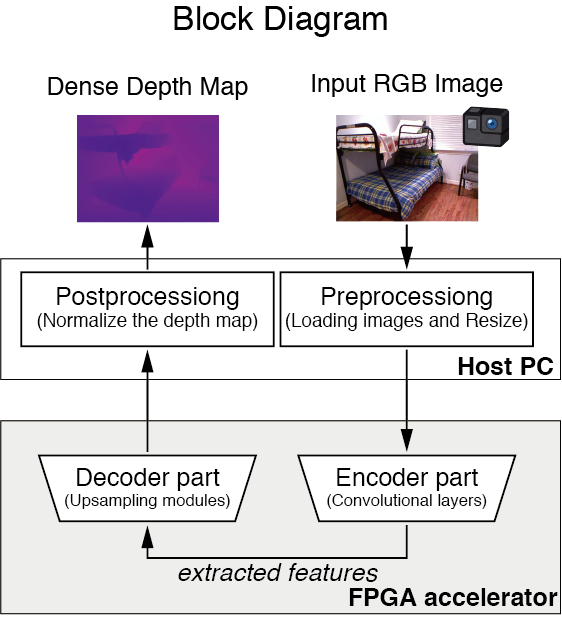This figure represents the block diagram of our monocular depth estimation. In the system, the host CPU performs light-weight pre-post processing, and the FPGA accelerates the cumbersome CNN part, which is consisted of the encoder and decoder. The encoder extracts feature from input image by 15 convolutional layers. Then, the decoder conducts a pixel-level prediction by 1x1 convolutional operations and up-sampling operations using bi-linear interpolation. Finally, the decoder output the estimated depth map, and it’s displayed on the host.

#### 3. Intel FPGA Virtues in Your Project

Requirement
The design needs to reach

• Realtime speed(29.1FPS)
• The minimal accuracy drops by our quantization and weight pruning technique, compared to its dense model

Virtues
Intel FPGA virtues in our system is as follows

• Energy efficient system by the custom hardware for the depth estimation using an FPGA
• Good expansion and performance with low-price PCI express connection
• Benefit of Intel CPUs; facilitation of the development using OpenCL, OpenCV and OpenGL for the host processing

a.Model Compression Technique

In this project, we adapt weight pruning and quantization for real-time processing and facilitating on-chip memory configuration. The two compression schemes are described below.

(1) Pruning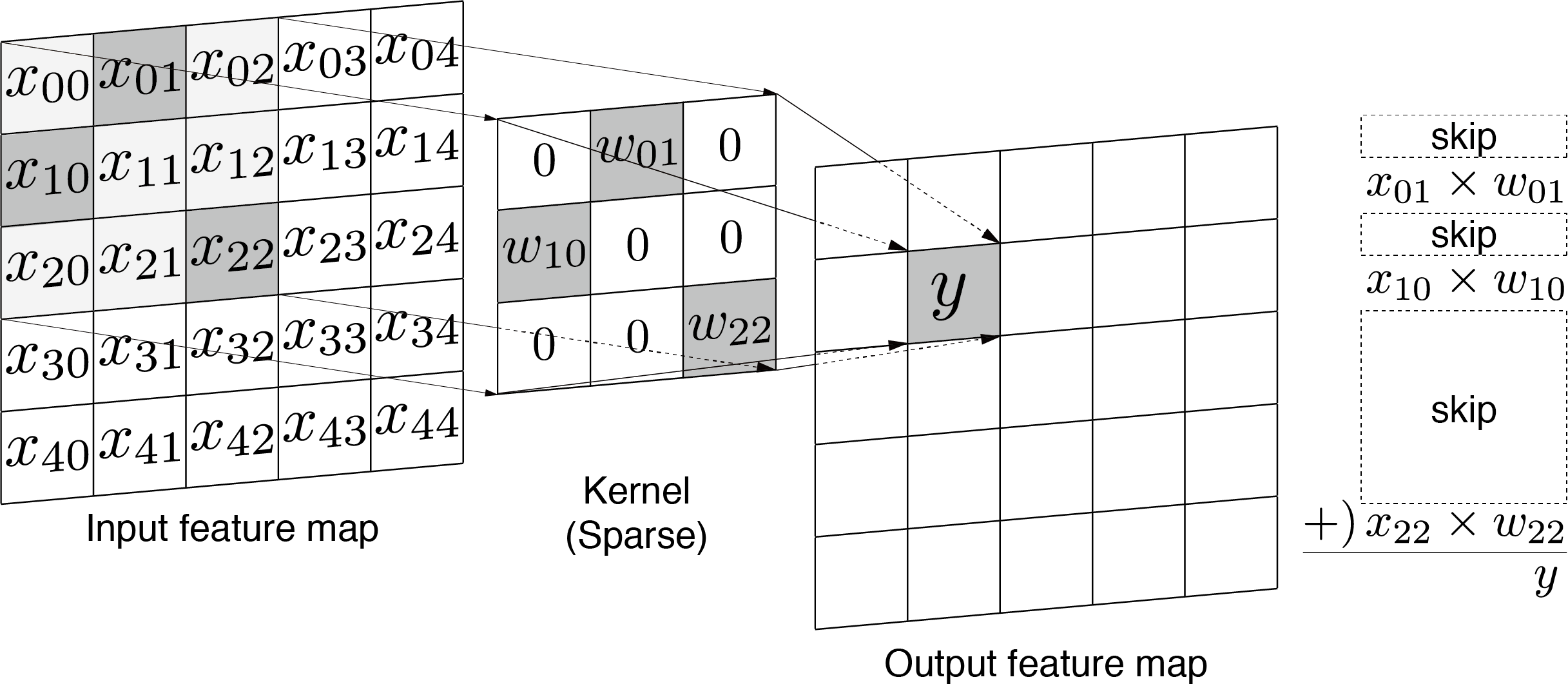Since the modern CNN requires a large number of weight parameters, an FPGA on-chip memories cannot store the all parameters. The above figure illustrates a sparse convolutional operation. After a pruning technique is applied, many weight values become zero. To store such weight parameters formed in the sparse matrix to block ROMs(M10Ks) efficiently, we employ coordinate(COO) format.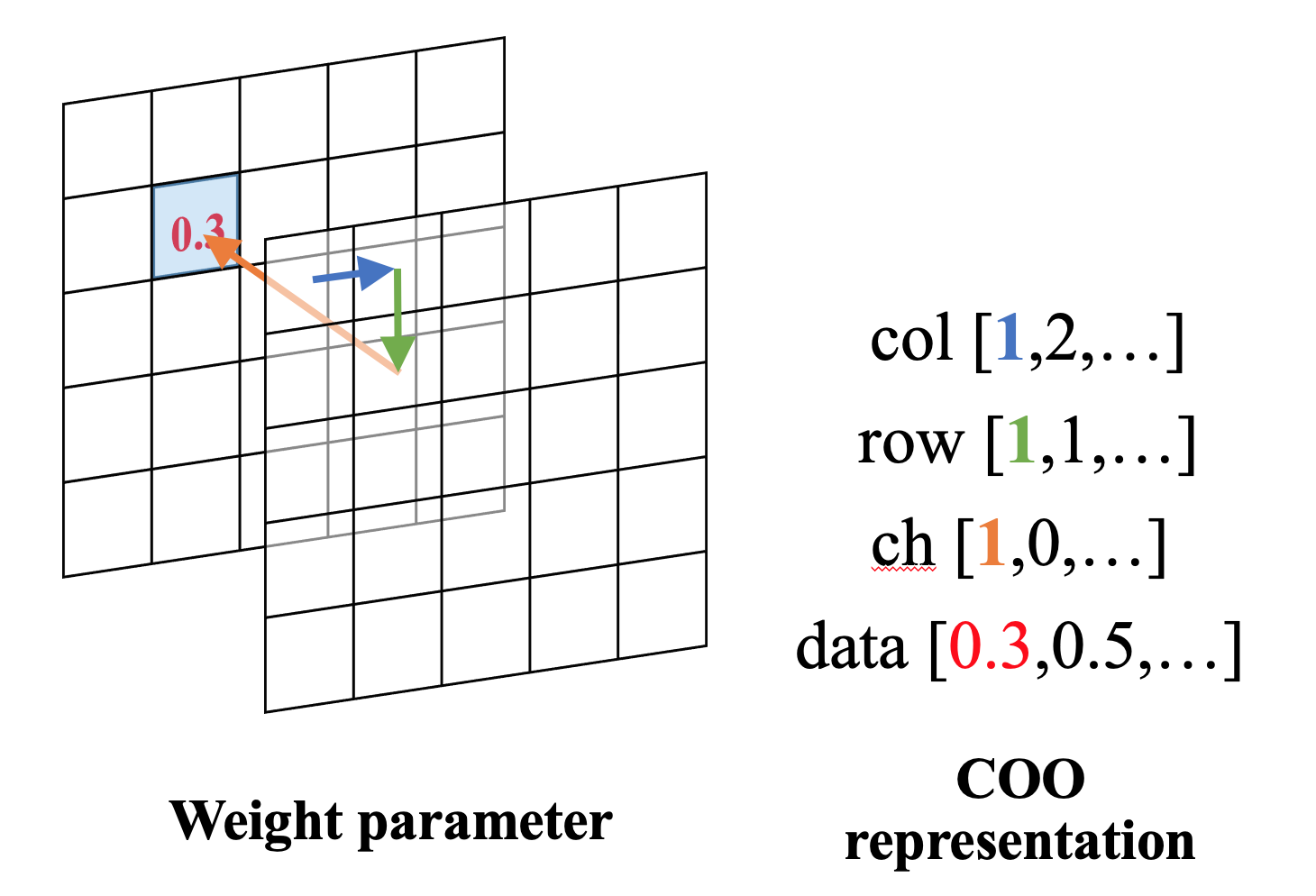The arrays, row, col, ch, and data store its row, column, channel indices, and nonzero weight values of the sparse matrix, respectively. When COO format is introduced, the sparse convolutional operation at (x, y) coordinates is formulated as follows. The dedicated circuit conducts MAC operations by skipping zero weight multiplications to realize high throughput.

(2) Quantization
Quantization in CNN is the representation of weights and/or activations with low bit precision, unlike a general float 32-bit precision. Based on the extensive research on low-bit (4-8-bit) CNN involving GPUs, CPUs and FPGAs, it is known that the low-bit representation accelerates convolutional operation with a minor reduction in the accuracy. Therefore, we chose 8-bit quantization for both the CNN weights and activations to reduce the redundant hardware area and to speed-up the operating frequency.

b. CNN model
We designed tiny Depth-CNN model based on separable convolution, which has a normal 3x3 convolutional layer and 14 separable convolutions. A separable convolution consists of depthwise convolution, which is the same operation as 2-dimensional convolution and point wise convolution, which is same as 1x1 conventional convolution. By converting the typical conventional KxK convolution to the separable convolution, the computational cost can be reduced. Typically, because K^2 << C_out (e.g., K=3 and C_out=256), where C_out denotes the number of output feature maps, the computational cost is reduced approximately by a factor of K^2 = 9.Here, the bottom table shows accuracy comparison of our Depth-CNN . The accuracy is measured with delta1 (the percentage of predicted pixels where the relative error is within 25%), and the error is RMSE (Root Mean Squared Error).D. Eigen, C. Puhrsch, and R. Fergus, “Depth map prediction from a single image using a multi-scale deep network,” in Advances in Neural Information Processing Systems (NIPS), 2014, pp. 2366–2374.

#### 5. Function Description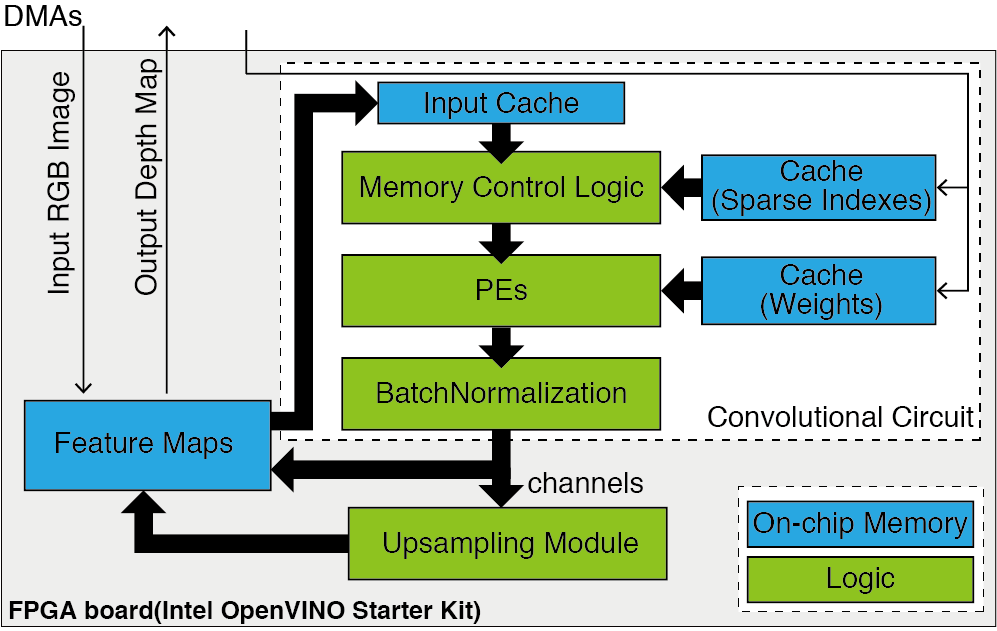This figure shows the functionality of our architecture. It realizes whole CNN calculation by performing convolutional operation layer by layer.　In our FPGA design, the single convolutional circuit handle general 3x3 convolutional operation, 3x3 depthwise convolutional one, 1x1 sparse convolutional one and 1x1 dense one. Our design performs many different convolutional operations with a one convolutional circuit so that endows low-power consumption and small-area. Considering the on-chip memory size, Feature maps are stored on single buffered on-chip memory, and CNN weights is stored on cache memory before the convolutional operation.

The key function in our architecture is the memory control logic. It handles the padding, the memory access with specified stride and indirect memory access depending on many different convolutional operations. Then PEs (Processing Elements) performs MAC operation of incoming input and CNN weights. To realize single buffered feature map, the input cache is adapted between the feature map memory and the memory control logic.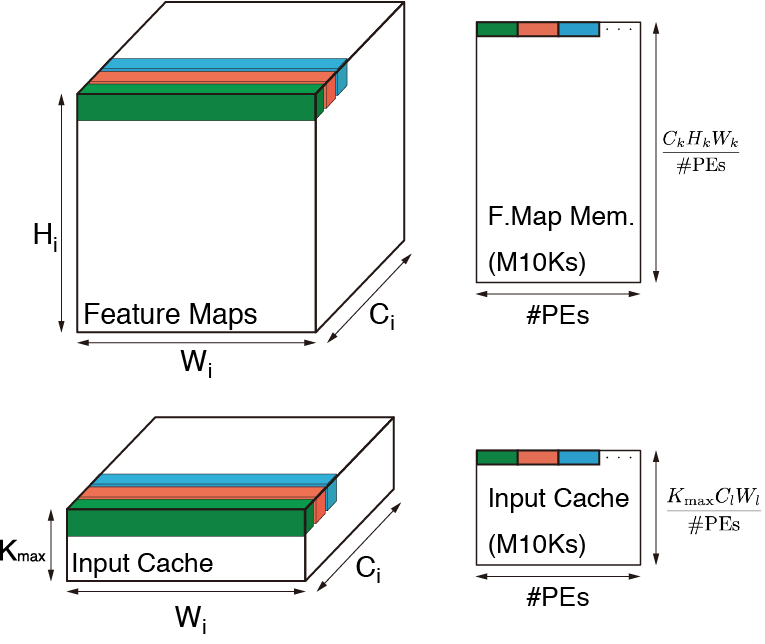This is the layout for parallel memory(M10K) access, where Hi, Ci and Wi denote the height, channel and width in the i-th layer, respectively. And Kmax indicates the maximum kernel size, k-th layer is the layer whose input feature map is the most large one (CkHkWk > CiHiWi, i\in{0,1,2,…}). Also, l-th layer takes most large ClWl. We set Kmax=3 and #PEs=128 for our CNN model. Because all Depth-CNN feature maps are 8-bit, and our memory layout is smaller than conventional double buffering or memory replicated design, all the feature maps are stored on the on-chip memory.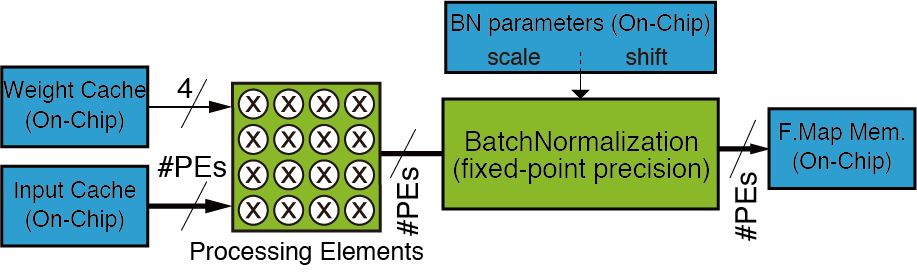This shows the PE and Batch Normalization Unit. The parameter M denotes the largest parallelism of channel-axis, and it is equal to #PEs/Wmin (=128/32=4 on our Depth-CNN). The four weights are broadcasted and PEs conduct multiply accumulations between input pixels and the weights.

#### 6. Performance Parameters

a. Experimental Setting

We develop the training scheme for the pruned and quantized CNN model using Chainer deep learning framework, and implement the FPGA acceralator using Intel FPGA SDK for OpenCL 17.1. The components of our FPGA platform are shown below:
-    OpenVINO starter kit
-    Intel(R) Core(TM) i7-8700K CPU @ 3.70GHz
-    Ubuntu 16.04, Intel FPGA SDK for OpenCL and OpenCV

We used NYUv2 dataset, which provides RGB and depth map images for the indoor scene. Our Depth-CNN is trained by 50,688 images and its accuracy and errors are evaluated by 654 test images. The following images show some inference results by our Depth-CNN on the test split.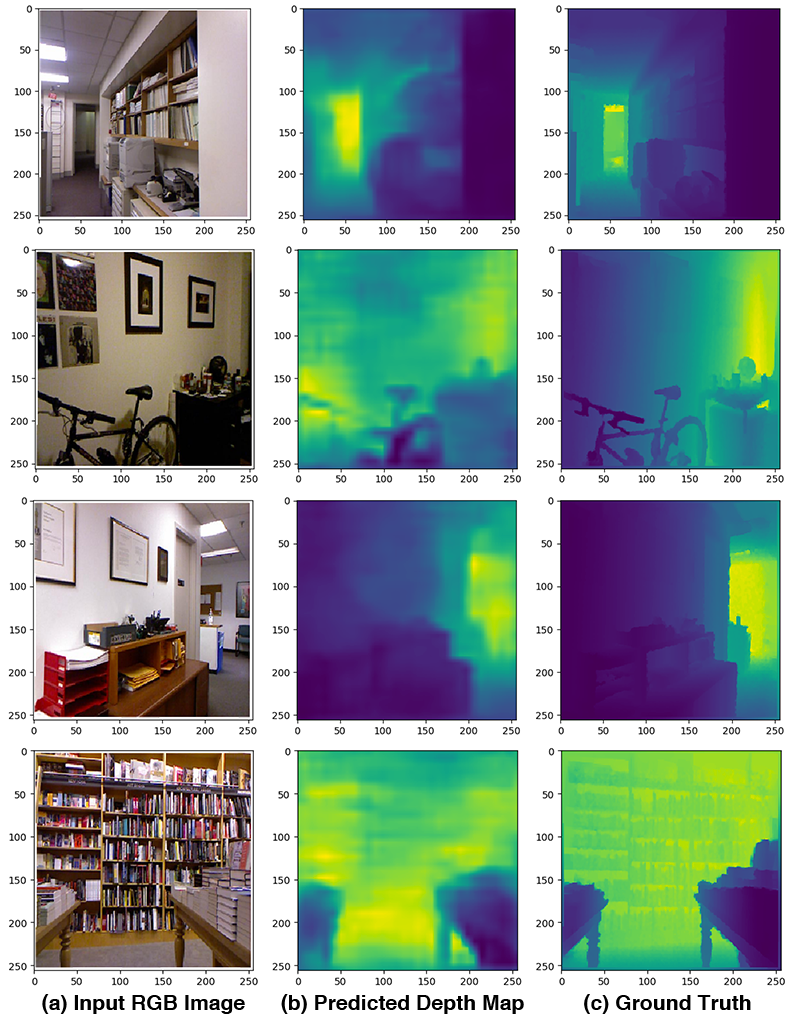b. Accuracy ComparisonThis shows accuracy comparison of our compression technique. As shown in the table, there is 1.6 points of negligible accuracy degradation, while the number of MACs is more than x5 fewer and CNN weights memory is x21 smaller.

b. Implementation Results
- Speed: 32.4 [FPS]
- Kernel fmax: 103.72 [Hz]
- #Registers: 165,637 ( 36 % )
- #ALMs: 70,210 ( 62 % )
- #DSPs: 224 ( 65 %)
- #RAM blocks (M10Ks): 1,009
Therefore, our implementation meets real-time speed.

#### 7. Design Architecture